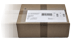# Casio FX-991ES PLUS 2nd edition

new
CHF 31.00When you order this item your purchase will be despatched free or charge
Availability
Available from stock  Delivery within 1-3 working days!
Manufacturer
Casio
Guarantee
36 Months
GTIN
4549526607066
Dimensions
76 x 160 x 13 mm
Weight
96 g
Directions for Use
German, French, Italian

#### Items delivered

The FX-991ES PLUS 2nd edition scientific calculator with 580 functions and new distribution functions is the most technically advanced model in the FX-ES Plus series making solving equations easy.
• 580 integrated functions
• The "natural display" enables you to enter fractions, square roots and other numerical expressions in the same way and display them exactly as they will appear in your textbook.
• Display: Natural-V.P.A.M., LCD (Dot-Matrix), 31 x 96 dots, 10 + 2 digits
• NEW: Distribution functions (Normal distribution and inverse normal distribution)
• NEW: Calculation of maximum and minimum of a function
• NEW: enhanced equation solver
• NEW: recurring decimal fractions
• NEW: Random integers
• NEW: statistical calculation of quartiles
• NEW: 40 Unit conversions
• Integral and differential calculus
• 40 physical constants
• 20 pairs of values for metric conversions
• Solving of equations (linear, high-order)
• 1 accumulative memory and 6 constant memories
• Automatic fraction calculator (two modes)
• Functions table
• Function calculation using the Calc key (temporary formula memory)
• SCI/FIX
• 24 levels of parentheses
• Repeat/correction function
• Numeric integration/numeric differentiation
• Base n calculations (BIN/OCT/DEC/HEX)
• Converts polar coordinates into rectangular coordinates and vice versa
• Calculation with complex numbers
• Matrix and vector operations
• Statistics (List-based statistics with 1 & 2 variables)
• Logical operations
• Permutations and combinatorics
• Random number generator
• Energy supply: Solar powered with an auxiliary battery
Accessories

^ top